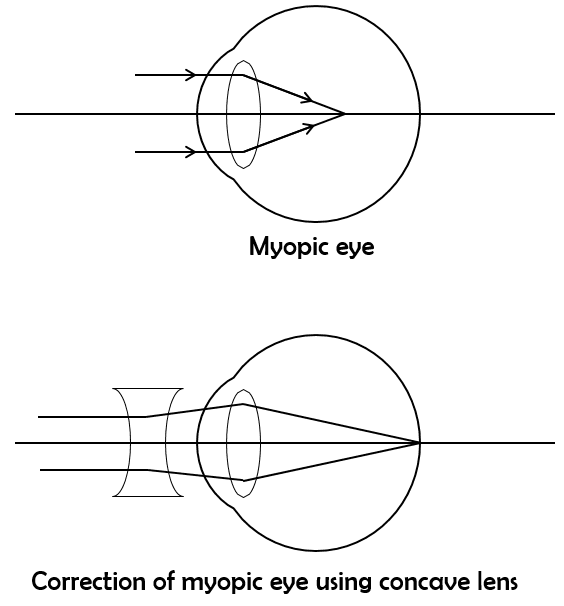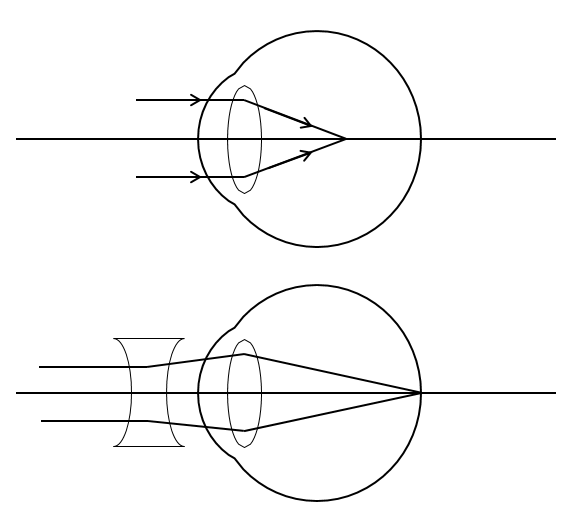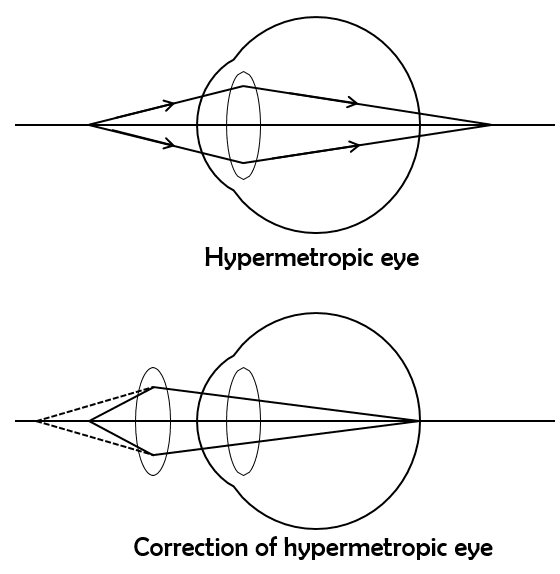# vs.eyeandcontacts.com

## Chapter 11 - The Human Eye and the Colorful World

Question 1: What is meant by the power of accommodation of the eye?
The ability of the eye lens to see both near and distant objects by adjusting its focal length is called the power of accommodation of the eye.

Question 2: A person with a myopic eye cannot see objects beyond 1.2 m distinctly. What should be the type of the corrective lens used to restore proper vision?
The person with a myopic eye who can’t see objects beyond 1.2 m distinctly should use concave lens with suitable power to restore proper vision.Question 3: What is the far point and near point of the human eye with normal vision?
The minimum distance at which the eye can see objects clearly is called the near point or least distance of distinct vision. For a normal eye it is 25cm. The farthest distance upto which the eye can see objects clearly is called the far point of the eye. For a normal eye it is between 25 cm and infinity.

Question 4: A student has difficulty reading the blackboard while sitting in the last row. What could be the defect the child is suffering from? How can it be corrected?
The student has difficulty in reading the blackboard while sitting in the last row which means that the child can see the near objects clearly while having difficulty in seeing the distant objects. Since the child can see the near objects clearly but has difficulty in seeing the distant object therefore that child is suffering from myopia. This defect of eye can be corrected using suitable concave lens.Question 1: The human eye can focus on objects at different distances by adjusting the focal length of the eye lens. This is due to
a) presbyopia
b) accommodation
c) near-sightedness
d) far-sightedness
b) Accommodation. Accommodation is the ability of the human eye to focus objects at different distances by adjusting the focal length of the eye lens.

Question 2: The human eye forms the image of an object at its
a) cornea
b) iris
c) pupil
d) retina
d) retina. The retina is a delicate membrane having enormous number of light-sensitive cells which get activated upon illumination and generate electrical signals. These signals are sent to the brain so that we can see.

Question 3: The least distance of distinct vision for a young adult with normal vision is about
a) 25 m
b) 2.5 cm
c) 25 cm
d) 2.5 m
c) 25 cm

Question 4: The change in focal length of an eye lens is caused by the action of the
a) pupil
b) retina
c) ciliary muscles
d) iris
c) ciliary muscles. The action of ciliary muscles causes changes in the focal length of the eye lens.

Question 5: A person needs a lens of power -5.5 dioptres for correcting his distant vision. For correcting his near vision he needs a lens of power +1.5 dioptre. What is the focal length of the lens required for correcting (i) distant vision, and (ii) near vision?

i) For distant vision,
Power, P = -5.5
Focal length, f = ?
Using P = 1/f
= P = 1/f
= -5.5 = 1/f
= -5.5 = 100/f
= -5.5f = 100
= f = 100/(-5.5)
= f = -18.18cm or f = -0.1818m
Therefore, the focal length of lens having power -5.5 is -0.18m or 18.18cm.

ii) For near vision,
Power, P = +1.5
Focal length, f = ?
Using P = 1/f
= P = 1/f
= +1.5 = 1/f
= +1.5 = 100/f
= +1.5f = 100
= f = 100/(+1.5)
= f = 66.67cm or f = 0.67m
Therefore, the focal length of lens having power +1.5 is 0.67m or 66.67cm.

Question 6: The far point of a myopic person is 80 cm in front of the eye. What is the nature and power of the lens required to correct the problem?
Since the person is suffering from myopia, therefore that person needs to use concave lens.

Now to find power of lens,
Object distance, u = infinity (∞)
Image distance, v = -80cm
Focal length, f = ?
Therefore,
1/f = 1/v - 1/u
1/f = 1/(-80) - 1/∞
1/f = 1/(-80)
f = -80cm
Power, P = 1/f
= P = 1/f
= P = 100/f
= P = 100/(-80)
= P = -1.25D

Hence a concave lens of power -1.25D is required by the person who is suffering from myopia.

Question 7: Make a diagram to show how hypermetropia is corrected. The near point of a hypermetropic eye is 1 m. What is the power of the lens required to correct this defect? Assume that the near point of the normal eye is 25 cm.
A person who is suffering from hypermetropia can see nearby object with difficulty while at the same time can see the distant object clearly. This defect occurs as the eye lens focuses the incoming divergent rays beyond retina. This defect can be corrected by using a suitable convex lens. The suitable convex lens converges the incoming light in such a way that the image is formed on the retina. The following image illustrates this.The convex lens creates a virtual image of a nearby object at near point of vision of the person who is suffering from hypermetropia. The person will be able to clearly see the object kept at 25cm (near point of the normal eye), if the image of the object is formed at his near point (1 meter).

Therefore, object distance, u = -25 cm
Image distance, v = -1 m or v = -100cm
Focal length, f = ?
Using lens formula:
1/v - 1/u = 1/f
= 1/(-100) - 1/(-25) = 1/f
= (-1 + 4)/100 = 1/f
= 3/100 = 1/f
= f = 100/3
= f = 33.33cm
Now, power, P = 1/f
= P = 100/f
= P = 100/33.33
= P = +3D

Therefore convex lens of power + 3.0D is required to correct the defect.

Question 8: Why is a normal eye not able to see clearly the objects placed closer than 25 cm?
A normal eye is unable to clearly see the objects placed closer than 25cm because the ciliary muscle on eyes are unable to contract beyond a certain limit.

Question 9: What happens to the image distance in the eye when we increase the distance of an object from the eye?
The image is formed on the retina even on increasing the distance of an object from the eye. For this, eye lens become thinner and its focal length increases as the object is moved away from the eye.

Question 10: Why do stars twinkle?
Stars twinkle due to atmospheric refraction of star light and due to the changing in the position of the stars and the movement of the layers of the atmosphere. So the light from the stars is sometimes brighter and sometimes fainter and it appears to twinkle. Planets are closer to the Earth than stars. The light from stars are considered as point source of light and the light from the planets are considered as extended source of light. So the light from planets nullify the twinkling effect.

Question 11: Explain why the planets do not twinkle.
Planets are much closer to the Earth and are seen as extended sources. So, a planet may be considered as a collection of a large number of point-sized light sources. Although light coming from individual point-sized sources flickers but the total amount of light entering our eye from all the individuals point-sized sources average out to be constant. Thereby, planets appear equally bright and there is no twinkling of planets.

Question 12: Why does the Sun appear reddish early in the morning?